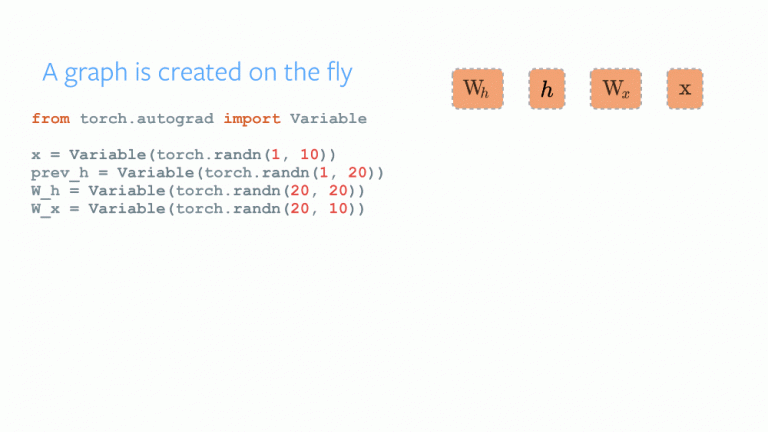@Team 2018-02-26T13:49:50.000000Z 字数 5876 阅读 1841

# PyTorch入门：一个简单但强大的深度学习库

叶虎

# 引言# 目录

• PyTorch概述
• PyTorch基础
• 使用Numpy和PyTorch实现一个神经网络
• 与其它深度学习库的对比
• 实战-使用PyTorch解决一个图像识别问题

# PyTorch概述

PyTorch的发明者们说他们的设计理念是命令式，这意味着可以立即运行计算。这契合Python的编程方式，我们不想等到实现所有代码后才能知道当前的代码是否有效，相反，我们可以非常容易运行一部分代码，并进行实时检查。对于神经网络模型的调试，这将是一个福音。

• 极简的API：和使用Python一样容易；
• 支持Python：像前面所说，PyTorch完美集成了Python的数据科学栈，使用它就和Numpy差不多；
• 动态计算图：PyTorch不是预先定义计算图，而是提供一个动态计算图，甚至在运行时可以改变它。当你不知道要为你的网络分配多大内存时，PyTorch就很有用。

PyTorch的另外优势是提供多GPU支持，而且提供规范的数据加载模块以及简单的预处理模块。

# PyTorch基础PyTorch的安装非常容易，你只需要按照官方文档说明在你的系统（目前不支持Windows）中运行特定的命令即可。下面是我所使用的命令：conda install pytorch torchvision cuda91 -c pytorch


• PyTorch张量
• 数学运算
• Optim模块
• nn模块

## PyTorch张量

# import pytorch
import torch

# 定义一个向量
torch.FloatTensor()
# 输出
2
[torch.FloatTensor of size 1]


## 数学运算

a = torch.FloatTensor()
b = torch.FloatTensor()
a + b
# 输出
5
[torch.FloatTensor of size 1]


matrix = torch.randn(3, 3)
matrix
-1.3531 -0.5394  0.8934
1.7457 -0.6291 -0.0484
-1.3502 -0.6439 -1.5652
[torch.FloatTensor of size 3x3]

matrix.t()
-2.1139  1.8278  0.1976
0.6236  0.3525  0.2660
-1.4604  0.8982  0.0428
[torch.FloatTensor of size 3x3]import torch
x.data
1  1
1  1
[torch.FloatTensor of size 2x2]

y = x + 2
z = y * y * 3
out = z.mean()
Variable containing:
4.5000  4.5000
4.5000  4.5000
[torch.FloatTensor of size 2x2]


## Optim模块

optimizer = torch.optim.Adam(model.parameters(), lr=learning_rate)


## nn模块

import torch

# 定义模型
model = torch.nn.Sequential(
torch.nn.Linear(input_num_units, hidden_num_units),
torch.nn.ReLU(),
torch.nn.Linear(hidden_num_units, output_num_units),
)
loss_fn = torch.nn.CrossEntropyLoss()


# 使用Numpy和PyTorch实现一个神经网络

# Numpy实现神经网络

import numpy as np

# 输入
X=np.array([[1,0,1,0],[1,0,1,1],[0,1,0,1]])

# 输出
y=np.array([,,])

# Sigmoid
def sigmoid (x):
return 1/(1 + np.exp(-x))

# Sigmoid导数
def derivatives_sigmoid(x):
return x * (1 - x)

# 参数设置
epoch=5000 #训练步长
lr=0.1 #学习速率
inputlayer_neurons = X.shape #特征数
hiddenlayer_neurons = 3 #隐含层神经元数
output_neurons = 1 #输出维度

#权重初始化
wh=np.random.uniform(size=(inputlayer_neurons,hiddenlayer_neurons))
bh=np.random.uniform(size=(1,hiddenlayer_neurons))
wout=np.random.uniform(size=(hiddenlayer_neurons,output_neurons))
bout=np.random.uniform(size=(1,output_neurons))

for i in range(epoch):
#前向过程
hidden_layer_input1=np.dot(X,wh)
hidden_layer_input=hidden_layer_input1 + bh
hiddenlayer_activations = sigmoid(hidden_layer_input)
output_layer_input1=np.dot(hiddenlayer_activations,wout)
output_layer_input= output_layer_input1+ bout
output = sigmoid(output_layer_input)

#反向过程
E = y-output
slope_output_layer = derivatives_sigmoid(output)
slope_hidden_layer = derivatives_sigmoid(hiddenlayer_activations)
d_output = E * slope_output_layer
Error_at_hidden_layer = d_output.dot(wout.T)
d_hiddenlayer = Error_at_hidden_layer * slope_hidden_layer
wout += hiddenlayer_activations.T.dot(d_output) *lr
bout += np.sum(d_output, axis=0,keepdims=True) *lr
wh += X.T.dot(d_hiddenlayer) *lr
bh += np.sum(d_hiddenlayer, axis=0,keepdims=True) *lr

print('actual :\n', y, '\n')
print('predicted :\n', output)


## pytorch实现网络
import torch

#输入
X = torch.Tensor([[1,0,1,0],[1,0,1,1],[0,1,0,1]])

#输出
y = torch.Tensor([,,])

#Sigmoid
def sigmoid (x):
return 1/(1 + torch.exp(-x))

#Sigmoid导数
def derivatives_sigmoid(x):
return x * (1 - x)

#参数设置
epoch=5000 #训练步长
lr=0.1 #学习速率
inputlayer_neurons = X.shape #输入维度
hiddenlayer_neurons = 3 #隐含层神经元数目
output_neurons = 1 #输出维度

#权重初始化
wh=torch.randn(inputlayer_neurons, hiddenlayer_neurons).type(torch.FloatTensor)
bh=torch.randn(1, hiddenlayer_neurons).type(torch.FloatTensor)
wout=torch.randn(hiddenlayer_neurons, output_neurons)
bout=torch.randn(1, output_neurons)

for i in range(epoch):

#前向过程
hidden_layer_input1 = torch.mm(X, wh)
hidden_layer_input = hidden_layer_input1 + bh
hidden_layer_activations = sigmoid(hidden_layer_input)

output_layer_input1 = torch.mm(hidden_layer_activations, wout)
output_layer_input = output_layer_input1 + bout
output = sigmoid(output_layer_input1)

#反向过程
E = y-output
slope_output_layer = derivatives_sigmoid(output)
slope_hidden_layer = derivatives_sigmoid(hidden_layer_activations)
d_output = E * slope_output_layer
Error_at_hidden_layer = torch.mm(d_output, wout.t())
d_hiddenlayer = Error_at_hidden_layer * slope_hidden_layer
wout += torch.mm(hidden_layer_activations.t(), d_output) *lr
bout += d_output.sum() *lr
wh += torch.mm(X.t(), d_hiddenlayer) *lr
bh += d_output.sum() *lr

print('actual :\n', y, '\n')
print('predicted :\n', output)


# 实战-使用PyTorch解决一个图像识别问题• 私有
• 公开
• 删除